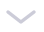### python中的值传递和引用传递

今天和大家分享python中很重要的一个知识点：参数传递，其中包括值传递和引用传递。

1.1 值传递

1.2 引用传递

# 1.1 值传递

``#include &lt;iostream&gt;using namespace std; // 交换2个变量的值void swap(int x, int y) {int temp;temp = x; // 交换x和y的值 x = y; y = temp; return;}int main () { int a = 1; int b = 2; cout &lt;&lt; &quot;Before swap, value of a :&quot; &lt;&lt; a &lt;&lt; endl; cout &lt;&lt; &quot;Before swap, value of b :&quot; &lt;&lt; b &lt;&lt; endl; swap(a, b);  cout &lt;&lt; &quot;After swap, value of a :&quot; &lt;&lt; a &lt;&lt; endl; cout &lt;&lt; &quot;After swap, value of b :&quot; &lt;&lt; b &lt;&lt; endl; return 0;}Before swap, value of a :1Before swap, value of b :2After swap, value of a :1After swap, value of b :2``

# 1.2 引用传递

``void swap(int&amp; x, int&amp; y) {   int temp;   temp = x; // 交换x和y的值   x = y;   y = temp;   return;}``

``Before swap, value of a :1Before swap, value of b :2After swap, value of a :2After swap, value of b :1``

# 二、Python变量及其赋值

``a = 1b = aa = a + 1``

``l1 = [1, 2, 3]l2 = l1l1.append(4)l1[1, 2, 3, 4]l2[1, 2, 3, 4]``

``l = [1, 2, 3]del l``

del l 删除了l这个变量，从此以后你无法访问l，但是对象[1, 2, 3]仍然存在。Python程序运行时，其自带的垃圾回收系统会跟踪每个对象的引用。如果[1, 2, 3]除了l外，还在其他地方被引用，那就不会被回收，反之则会被回收。

• 变量的赋值，只是表示让变量指向了某个对象，并不表示拷贝对象给变量；而一个对象，可以被多个变量所指向。

• 可变对象（列表，字典，集合等等）的改变，会影响所有指向该对象的变量。

• 对于不可变对象（字符串、整型、元组等等），所有指向该对象的变量的值总是一样的，也不会改变。但是通过某些操作（+=等等）更新不可变对象的值时，会返回一个新的对象。

• 变量可以被删除，但是对象无法被删除。

# 三、Python函数的参数传递

``def my_func1(b):	b = 2 a = 1my_func1(a)a1``

``def my_func2(b):	b = 2	return b a = 1a = my_func2(a)a2``

``def my_func3(l2):	l2.append(4) l1 = [1, 2, 3]my_func3(l1)l1[1, 2, 3, 4]``

``def my_func4(l2):	l2 = l2 +  l1 = [1, 2, 3]my_func4(l1)l1[1, 2, 3]``

``def my_func5(l2):	l2 = l2 + 	return l2 l1 = [1, 2, 3]l1 = my_func5(l1)l1[1, 2, 3, 4]``

• my_func3()中单纯地改变了对象的值，因此函数返回后，所有指向该对象的变量都会被改变。

• 但my_func4()中则创建了新的对象，并赋值给一个本地变量，因此原变量仍然不变。

# 四、总结

• 如果对象是可变的，当其改变时，所有指向这个对象的变量都会改变。

• 如果对象不可变，简单的赋值只能改变其中一个变量的值，其余变量则不受影响。